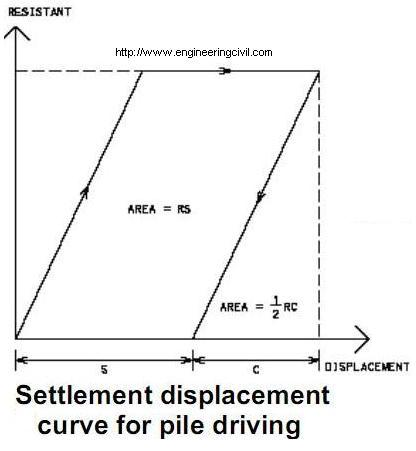Search

# In Hiley’s formula for driven piles, why is a coefficient of 0.5 applied for the term elastic deformation of piles and soil?

Posted in Piles Engineering |Email This Post |

Hiley’s formula R=E/(s+0.5c) is based on the principle of energy conservation in which the energy brought about by hammers during the action of hitting are transferred to piles in ground. When the hammer force and displacement is plotted, the energy absorbed by piles is the area under the curve.

Since the curve of elastic component is linear with a positive slope, the area i.e. energy should be the area of triangle (0.5xRxc) where R is reaction force and c is elastic compression due to helmet, piles and soil system. For settlement, it is of horizontal line in force-displacement diagram and hence the energy transferred to pile-soil system is (Rxs).